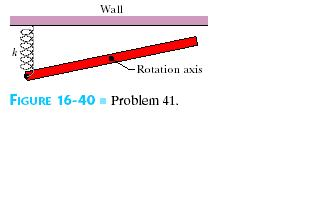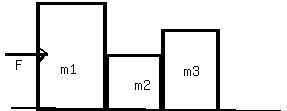Ask question

# A 0.30 kg ladle sliding on a horizontal frictionless surface isattached to one end of a horizontal spring (k = 500 N/m) whoseother end is fixed. The ladle has a kinetic energy of 10 J as itpasses through its equilibrium position (the point at which thespring force is zero). (a) At what rate is the spring doing work on the ladle as the ladlepasses through its equilibrium position? (b) At what rate is the spring doing work on the ladle when thespring is compressed 0.10 m and the ladle is moving away from theequilibrium position?# A 0.30 kg ladle sliding on a horizontal frictionless surface isattached to one end of a horizontal spring (k = 500 N/m) whoseother end is fixed. The ladle has a kinetic energy of 10 J as itpasses through its equilibrium position (the point at which thespring force is zero). (a) At what rate is the spring doing work on the ladle as the ladlepasses through its equilibrium position? (b) At what rate is the spring doing work on the ladle when thespring is compressed 0.10 m and the ladle is moving away from theequilibrium position?

Question
Otherasked 2021-02-23
A 0.30 kg ladle sliding on a horizontal frictionless surface isattached to one end of a horizontal spring (k = 500 N/m) whoseother end is fixed. The ladle has a kinetic energy of 10 J as itpasses through its equilibrium position (the point at which thespring force is zero).
(a) At what rate is the spring doing work on the ladle as the ladlepasses through its equilibrium position?
(b) At what rate is the spring doing work on the ladle when thespring is compressed 0.10 m and the ladle is moving away from theequilibrium position?

## Answers (1)2021-02-25
(a)Since the force exertedby the spring on the mass 0.30 kg is zero when the mass passesthrough the equilibrium position of the spring, the rate at whichthe spring is doing work on the mass 0.30 kg at this instant isalso zero.
(b)Force (F) = k x
= 500 * 0.1 N
= 50.0 N
Apply, Law of conservation of energy:
$$\displaystyle{10.0}{J}={\left(\frac{{1}}{{2}}\right)}{m}{v}^{{{2}}}+{\left(\frac{{1}}{{2}}\right)}{k}{x}^{{{2}}}$$
or v = 7.1 m/s
rate is the spring doing work on the ladle when the springis compressed 0.10m
P = - F . v
= 50.0 * 7.1 W
$$\displaystyle{P}=-{3.5}\cdot{10}^{{{2}}}{W}$$

### Relevant Questionsasked 2021-03-24
A 2.4-kg object is attached to a horizontal spring of forceconstant k=4.5 kN/m. The spring is stretched 10 cm fromequilibrium and released. Find (a) the frequency of themotion, (b) the period, (c) the amplitude, (d) the maximum speed,and (e) the maximum acceleration. (f) When does the objectfirst reach its equilibrium position? What is itsacceleration at this time?
Two identical blocks placed one on top of the other rest on africtionless horizontal air track. The lower block isattached to a spring of spring constant k= 600 N/m. Whendisplaced slightly from its equilibrium position, the systemoscillates with a frequency of 1.8 Hz. When the amplitude ofoscillation exceeds 5 cm, the upper block starts to slide relativeto the lower one. (a) What are the masses of the twoblocks? (b) What is the coefficient of static frictionbetween the two blocks?asked 2021-02-19
A 10 kg objectexperiences a horizontal force which causes it to accelerate at 5 $$\displaystyle\frac{{m}}{{s}^{{2}}}$$, moving it a distance of 20 m, horizontally.How much work is done by the force?
A ball is connected to a rope and swung around in uniform circular motion.The tension in the rope is measured at 10 N and the radius of thecircle is 1 m. How much work is done in one revolution around the circle?
A 10 kg weight issuspended in the air by a strong cable. How much work is done, perunit time, in suspending the weight?
A 5 kg block is moved up a 30 degree incline by a force of 50 N, parallel to the incline. The coefficient of kinetic friction between the block and the incline is .25. How much work is done by the 50 N force in moving the block a distance of 10 meters? What is the total workdone on the block over the same distance?
What is the kinetic energy of a 2 kg ball that travels a distance of 50 metersin 5 seconds?
A ball is thrown vertically with a velocity of 25 m/s. How high does it go? What is its velocity when it reaches a height of 25 m?
A ball with enough speed can complete a vertical loop. With what speed must the ballenter the loop to complete a 2 m loop? (Keep in mind that the velocity of the ball is not constant throughout the loop).asked 2021-05-10
In the overhead view of, a long uniform rod of mass m0.6 Kg is free to rotate in a horizontal planeabout a vertical axis through its center .A spring with force constant k = 1850 N/m is connected horizontally betweenone end of the rod and a fixed wall. When the rod is in equilibrium, it is parallel to the wall. What isthe period of the small oscillations thatresult when the rod is rotated slightly and released?asked 2021-04-18
A 2.0-kg projectile is fired with initial velocity components $$\displaystyle{v}_{{{0}{x}}}={30}$$ m/s and $$\displaystyle{v}_{{{0}{y}}}={40}$$ m/s from a point on the earth's surface. Neglect any effects due to air resistance. What is the kinetic energy of the projectile when it reaches the highest point in its trajectory? How much work was done in firing the projectile?asked 2021-04-13
As depicted in the applet, Albertine finds herself in a very odd contraption. She sits in a reclining chair, in front of a large, compressed spring. The spring is compressed 5.00 m from its equilibrium position, and a glass sits 19.8m from her outstretched foot.
a)Assuming that Albertine's mass is 60.0kg , what is $$\displaystyle\mu_{{k}}$$, the coefficient of kinetic friction between the chair and the waxed floor? Use $$\displaystyle{g}={9.80}\frac{{m}}{{s}^{{2}}}$$ for the magnitude of the acceleration due to gravity. Assume that the value of k found in Part A has three significant figures. Note that if you did not assume that k has three significant figures, it would be impossible to get three significant figures for $$\displaystyle\mu_{{k}}$$, since the length scale along the bottom of the applet does not allow you to measure distances to that accuracy with different values of k.asked 2021-02-21
A 15.0 kg block is dragged over a rough, horizontal surface by a70.0 N force acting at 20.0 degree angle above the horizontal. The block is displaced 5.0 m, and the coefficient of kinetic friction is 0.3. Find the work done on the block by ; a) the 70.0 N force,b) the normal force, and c) the gravitational force. d) what is the increase in the internal energy of the block-surface system due to friction? e) find the total change in the kinetic energy of the block.asked 2021-05-20
Assume that a ball of charged particles has a uniformly distributednegative charge density except for a narrow radial tunnel throughits center, from the surface on one side to the surface on the opposite side. Also assume that we can position a proton any where along the tunnel or outside the ball. Let $$\displaystyle{F}_{{R}}$$ be the magnitude of the electrostatic force on the proton when it islocated at the ball's surface, at radius R. As a multiple ofR, how far from the surface is there a point where the forcemagnitude is 0.44FR if we move the proton(a) away from the ball and (b) into the tunnel?asked 2021-04-25
The unstable nucleus uranium-236 can be regarded as auniformly charged sphere of charge Q=+92e and radius $$\displaystyle{R}={7.4}\times{10}^{{-{15}}}$$ m. In nuclear fission, this can divide into twosmaller nuclei, each of 1/2 the charge and 1/2 the voume of theoriginal uranium-236 nucleus. This is one of the reactionsthat occurred n the nuclear weapon that exploded over Hiroshima, Japan in August 1945.
A. Find the radii of the two "daughter" nuclei of charge+46e.
B. In a simple model for the fission process, immediatelyafter the uranium-236 nucleus has undergone fission the "daughter"nuclei are at rest and just touching. Calculate the kineticenergy that each of the "daughter" nuclei will have when they arevery far apart.
C. In this model the sum of the kinetic energies of the two"daughter" nuclei is the energy released by the fission of oneuranium-236 nucleus. Calculate the energy released by thefission of 10.0 kg of uranium-236. The atomic mass ofuranium-236 is 236 u, where 1 u = 1 atomic mass unit $$\displaystyle={1.66}\times{10}^{{-{27}}}$$ kg. Express your answer both in joules and in kilotonsof TNT (1 kiloton of TNT releases 4.18 x 10^12 J when itexplodes).asked 2021-03-22
A box is sliding with a speed of 4.50 m/s on a horizontal surface when, at point P, it encounters a rough section. On the rough section, the coefficient of friction is not constant but starts at .100 at P and increases linerly with distance past P, reaching a value of .600 at 12.5 m past point P. (a) Use the work energy theorem to find how far this box slides before stopping. (b) What is the coefficient of friction at the stopping point? (c) How far would the box have slid iff the friciton coefficient didn't increase, but instead had the constant value of .1?asked 2021-03-24
The figure shows 3 crates being pushed over a concrete floor by a horizontal force f of magnitude 440N. The masses of the cratesare $$\displaystyle{m}_{{1}}={30}$$ kg, $$\displaystyle{m}_{{2}}={10}$$ kg, and $$\displaystyle{m}_{{3}}={20}$$ kg.The coefficient of kineticfriction between the floor and each of the crates is 0.7. a) what is the magnitude $$\displaystyle{F}_{{{32}}}$$ of the force on crate 3 from crate 2? b) If the crates then slide onto a polished floor, where the coefficientof kinetic friction is less than 0.700, is magnitude PSKF_{32}ZSL more than,less than, or the same as it was when the coeffient was 0.700?...